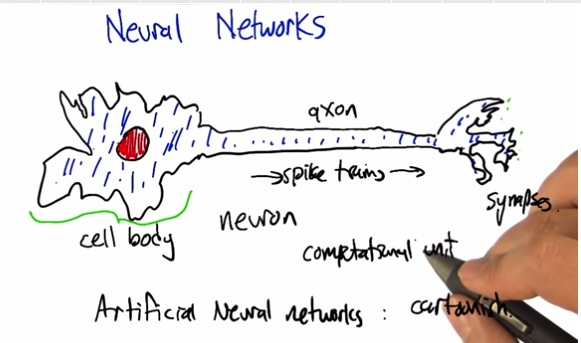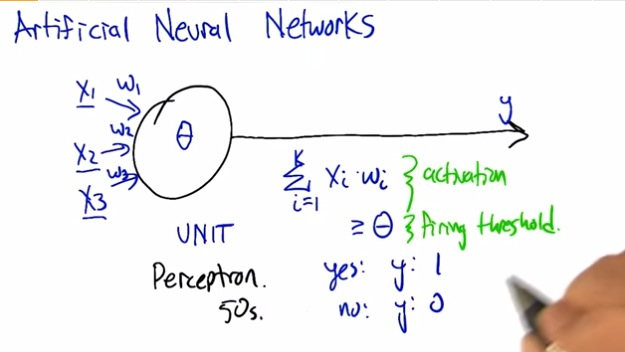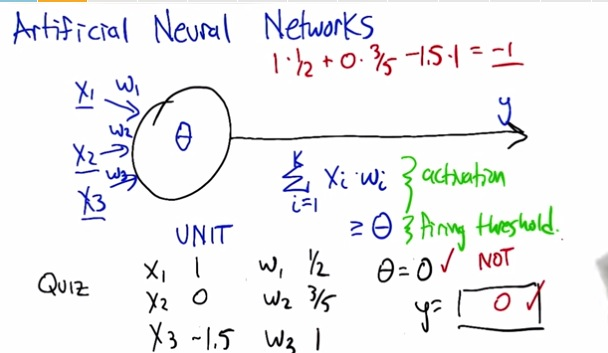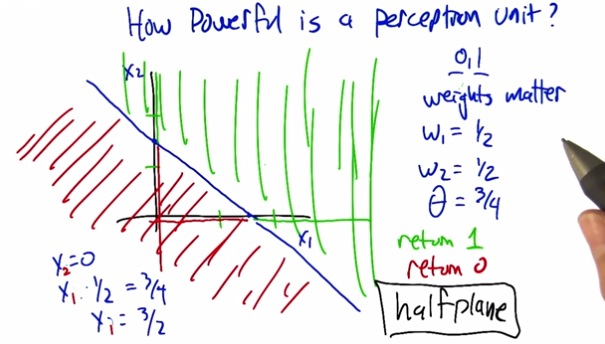• So we're creating truth table from this one.
• For example if x1 and x2 is either 1 or 0, and both weights are 1/2. Suppose one equals zero, what are the value of the others to be at least equals the threshold?
• We con do some equation, either x1 or x2 are zero. and create the point.
• The result will be a plane that drawn to separate the y=0/y=1 threshold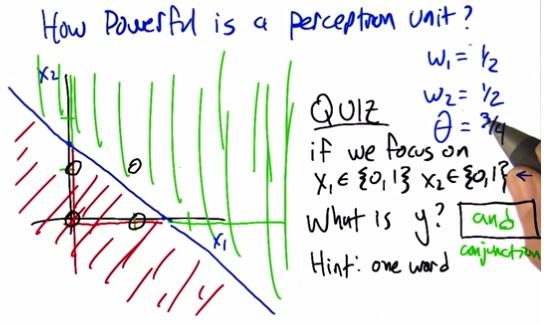• We have specified the line separator for the plane. And if we plot x1,x2 like truth table. We ended up to must have both of them equals 1 to be in the green zone. And that's makes an "AND" table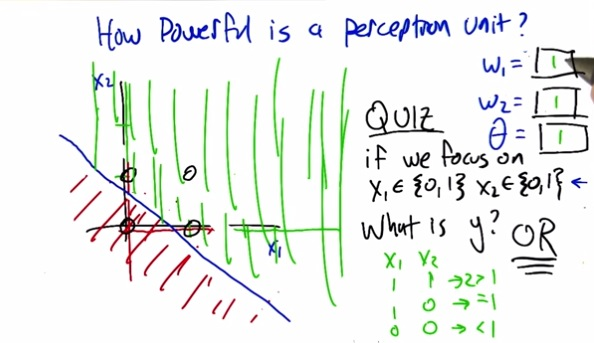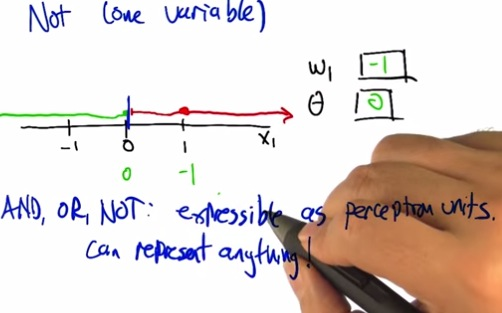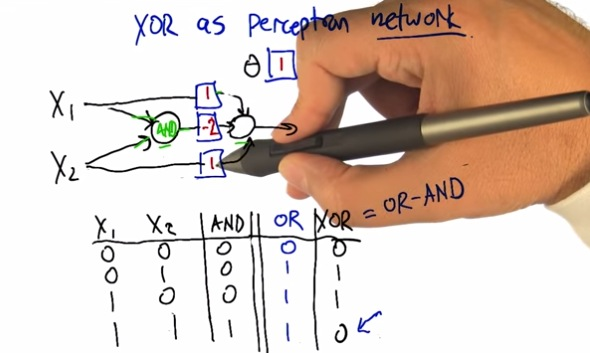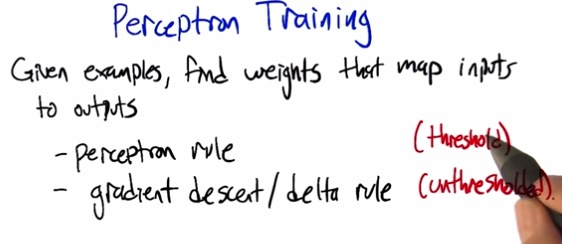• Perceptron training is training our perceptron. It will be wastefull to just do perceptron by hand.
• There's two options, perceptron rule or gradient descent.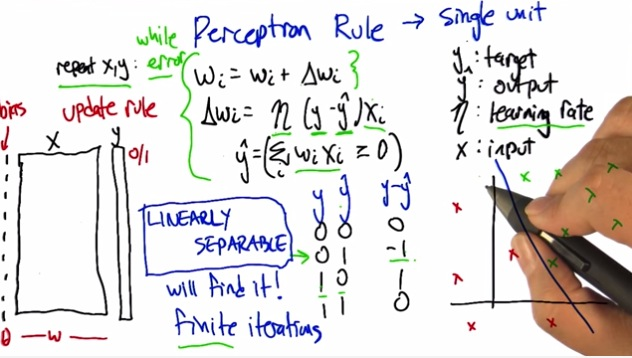• Now what we do is some kind of other supervised learning, where we structure our data to have x data set and coressponding parameters, with the output as our data target.
• Then we perform iteration in curly braces. Each weight  will be increasing every iterations.
• The delta weight will be learning rate . (y-h(x) . x data set
• here, the H(x) is the activation unit, and the threshold got subtittute to the left side as bias unit.
• If we cand find linear separable from the data, then the neural networks will find it.
• Otherwise it goes to infinite loop.